# Catch up on the latest AI articles# Techniques For Training GANs On A Small Number Of Data

3 main points
✔️ Successful fine-tuning of GAN with only 10 cards
✔️ Fischer information is important
✔️ Elastic weight consolidation is effective for GAN

written by Yijun LiRichard ZhangJingwan LuEli Shechtman
(Submitted on 4 Dec 2020)

Subjects: Computer Vision and Pattern Recognition (cs.CV)code：## First of all

In recent years, many GAN learning theories have been published, and it is becoming a thing of the past that "GANs cannot be learned without large data"; AI-SCHOLAR also has an article on learning GANs with a small amount of data. ↓

If you read these two articles and this article, I think you can catch up with the learning system with a small number of data which is the focus of attention in GAN. The paper I'm going to introduce here is a paper that claims to have succeeded in fine-tuning a GAN with only about 10 pieces of data. The following figure shows what we want to do.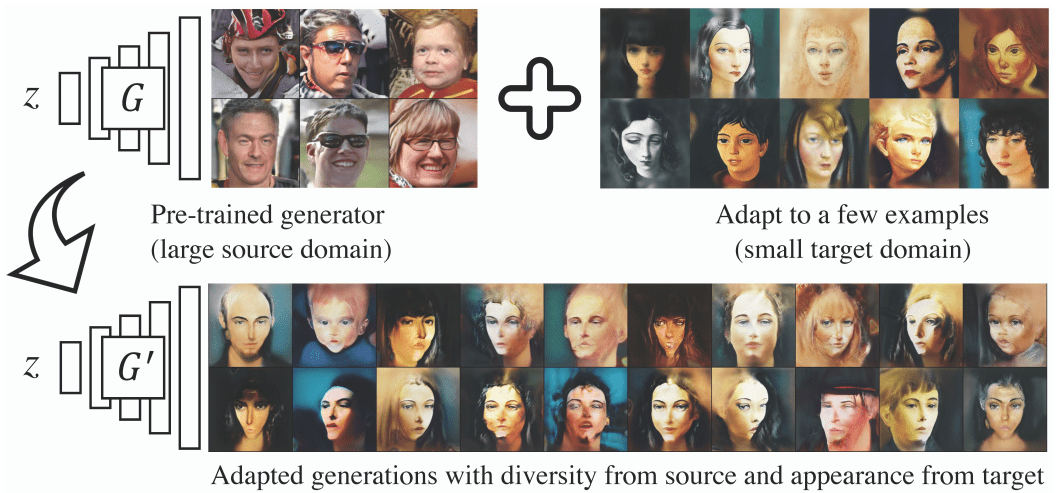## Proposed method

It is basically difficult to do fine-tuning with a small number of data. It is unreasonable to think that it is possible to completely infer the distribution of a small number of data. It is the same problem as it is obviously difficult to infer a curve from a single point. In such a case, it is natural to think of using a source domain that has been previously learned. However, in general, if you let the transfer learning happen, the target domain is good, but the performance in the However, in general, transfer learning results in a good performance in the target domain but dramatically poor performance in the source domain (this phenomenon is called CatastrophicForgetting). (This phenomenon is called Catastrophic Forgetting.) So even if you try to use the source domain, it won't work.

So our main technique is elastic weight consolidation (continuous learning). Continuous learning is similar to transfer learning but in a slightly different framework. Continuous learning has already been explained in the AI-SCHOLAR article, so please check this article. →( Learning Continuous Learning. Meta-learning for selective plasticity and the prevention of catastrophic forgetting! )

In this article, we will discuss how to apply elastic weight consolidation to GANs and how it can be used to learn small numbers of data, which is difficult in GANs.

### Elastic weight consolidation

The authors assume that the target domain has a We assume that there is a wealth of data on which to train generative models, and we conduct experiments to gain inspiration on what good weights might look like.

We use a large scale We collect the source data and the large scale target data and measure the rate of change of the weights using the 5-layer DCGAN. The result is shown in the following figure.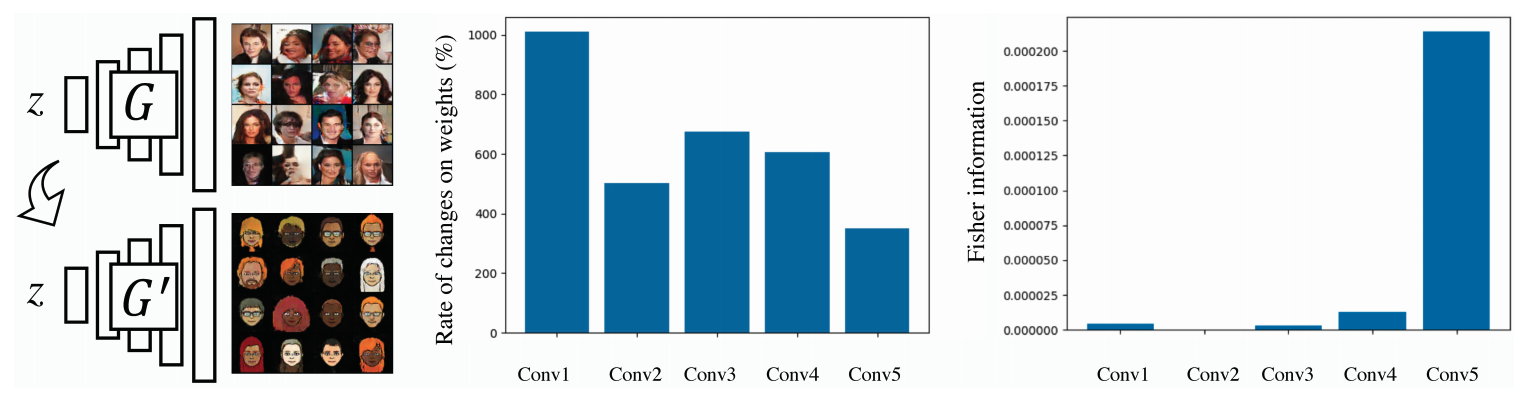I found something important here! That is the weights in the last layer (Conv5) are changing at a smaller rate than Conv1. The source data-learned weights in the initial layer are not important because they have changed significantly to learn the target domain, and the final layer holds more important weights for both the source and target data. It is possible to increase the success rate of fine-tuning by making it easier to retain the important weights of the final layer, instead of simply fine-tuning. This means that the weights of different layers should be regularized in different ways.

The next question is how to quantify or measure the importance of each weight. If you have a good intuition, you will notice this. Recall that in mathematical statistics, the Fisher information F can indicate how well the parameters of a model can be estimated given the observed values. (A Tutorial on Fisher information )

Given a pre-trained generative model for the source domain, the Fisher information $F$ can be computed by generating a certain amount of data $X$ given the training values of the network parameters $θ_s$ as follows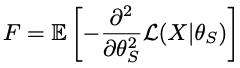$L(X|θ_s)$ is the log-likelihood function, which is equivalent to computing the binary cross-entropy loss using the output of the discriminator.

Just for simplicity, we use the output of the discriminator and show the average F of the weights in the different layers of the G model trained on real face images on the right side of the above figure. Clearly, we can see that the weights in the last layer have a much higher $F$ than the weights in the other layers. Considering the rate of change of the weights, we can directly use $F$ as a measure of the importance of the weights and add a regularization loss to penalize the change of the weights during adaptation to the target region.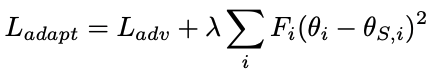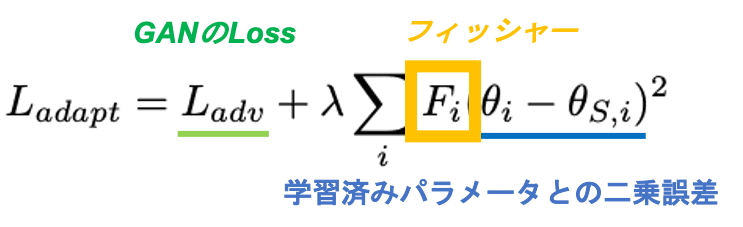So, save $F$ after pre-learning, and use $F$ for regularization during fine-tuning.

## Qualitative evaluation

We will see a comparison of the actual generation results between NST, BSA, and MineGAN, fine-tuning the FFHQ-trained model with 10 Emoji data.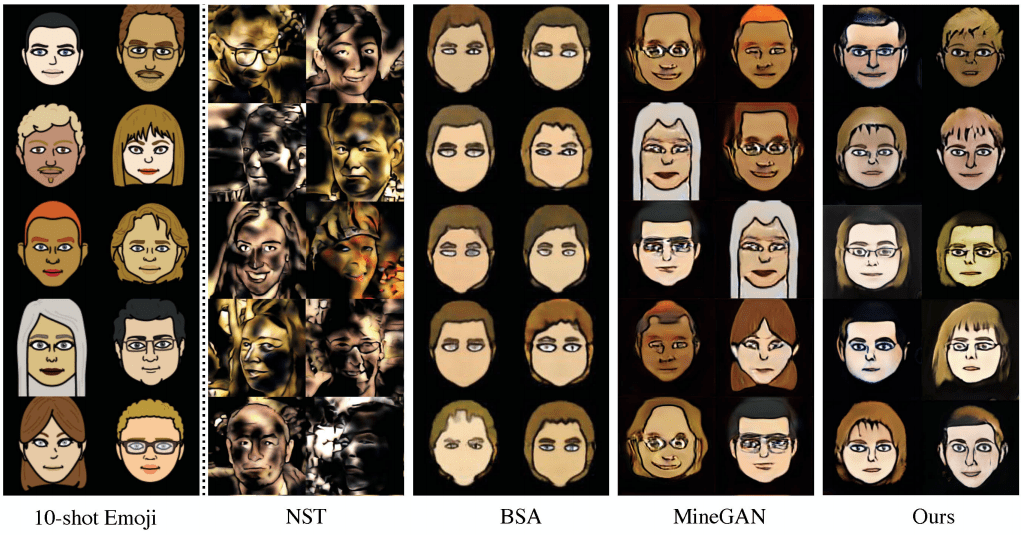The results are clear. MineGAN also seems to be able to generate, but it is clearly overtrained. The proposed method is able to inherit diversity from the source because it generates images that are not in the training data.

Experiments also showed that the larger the gap between the source and target domains, the less well it works.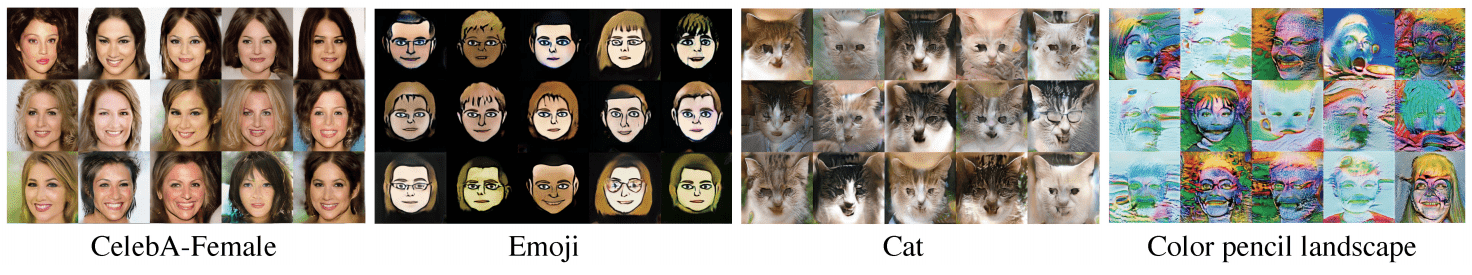CelebA-Female and Emoji are working relatively well because the gap is small, but Color pencil landscape is not generating properly because the gap is too large.

## Quantitative evaluation

We also evaluate FID, LPIPS, and User's subjective evaluation (the error rate of the generated image in the image pair) when fine-tuning 10 images.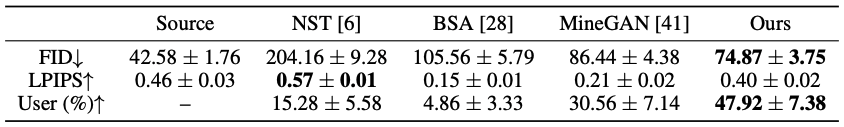You can see the high accuracy in comparison. In addition, the accuracy is much higher than other methods in User evaluation.

## Summary

We have established a successful method for fine-tuning GANs on a small number of data, and Elastic weight consolidation works well for training GANs, with overwhelming accuracy compared to other methods. The method is also quite simple and easy to understand.

If you have any suggestions for improvement of the content of the article,